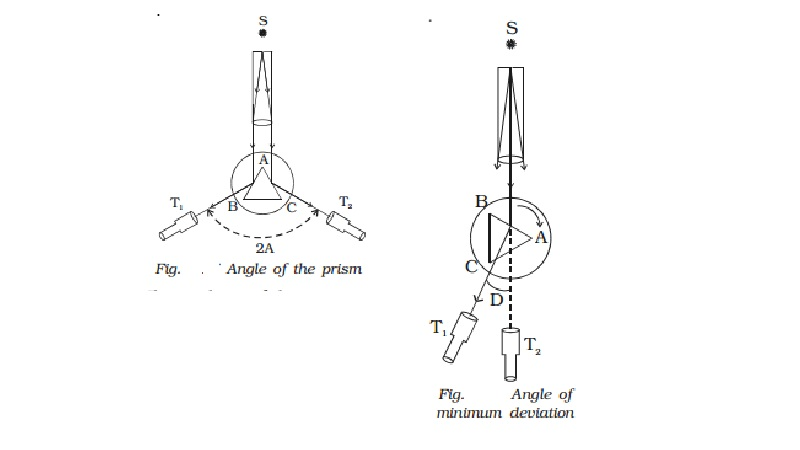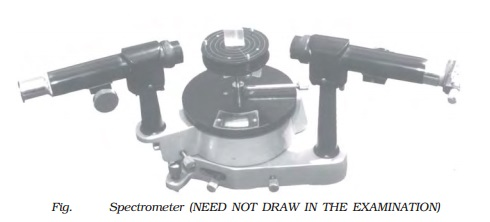Home | | Physics | Determination of the refractive index of the material of the prism

# Determination of the refractive index of the material of the prismThe preliminary adjustments of the telescope, collimator and the prism table of the spectrometer are made. The refractive index of the prism can be determined by knowing the angle of the prism and the angle of minimum deviation.Determination of the refractive index of the material of the prism

The preliminary adjustments of the telescope, collimator and the prism table of the spectrometer are made. The refractive index of the prism can be determined by knowing the angle of the prism and the angle of minimum deviation.

(i) Angle of the prism (A)

The prism is placed on the prism table with its refracting edge facing the collimator as shown in Fig . The slit is illuminated by a sodium vapour lamp.The parallel rays coming from the collimator fall on the two faces AB and AC.

The telescope is rotated to the position T1  until the image of the slit, formed by the reflection at the face AB is made to coincide

with the vertical cross wire of the telescope. The readings of the verniers are noted. The telescope is then rotated to the position T2  where the image of the slit formed by the reflection at the face AC coincides with the vertical cross wire. The readings are again noted.

The difference between these two readings gives the angle rotated by the telescope. This angle is equal to twice the angle of the prism. Half of this value gives the angle of the prism A.

(ii) Angle of minimum deviation (D)

The prism is placed on the prism table so that the light from the collimator falls on a refracting face, and the refracted image is observed through the telescope (Fig. ). The prism table is now rotated so that the angle of deviation decreases. A stage comes when the image stops for a moment and if we rotate the prism table further in the same direction, the image is seen to recede and the angle of deviation increases. The vertical cross wire of the telescope is made to coincide with the image of the slit where it turns back. This gives the minimum deviation position. The readings of the verniers are noted. Now the prism is removed and the telescope is turned to receive the direct ray and the vertical cross wire is made to coincide with the image. The readings of the verniers are noted. The difference between the two readings gives the angle of minimum deviation D.

The refractive index of the material of the prism ? is calculated using the formula ? = sin((A+D) / 2) / sin(A/2)

The refractive index of a liquid may be determined in the same way using a hollow glass prism filled with the given liquid.Study Material, Lecturing Notes, Assignment, Reference, Wiki description explanation, brief detail
11th 12th std standard Class Physics sciense Higher secondary school College Notes : Determination of the refractive index of the material of the prism |